# Cepheid

Cepheids are a type of star in which their brightness varies constantly and periodically with time. Not all Cepheids have the same brightness, but they do all share a very important characteristic: their luminosity is directly related to their period of variation. Thus, the brighter stars have longer cycles of variation; and the less bright, have shorter periods. And always, they are directly proportional. Therefore, by measuring its period we already know what the intrinsic brightness of that star is.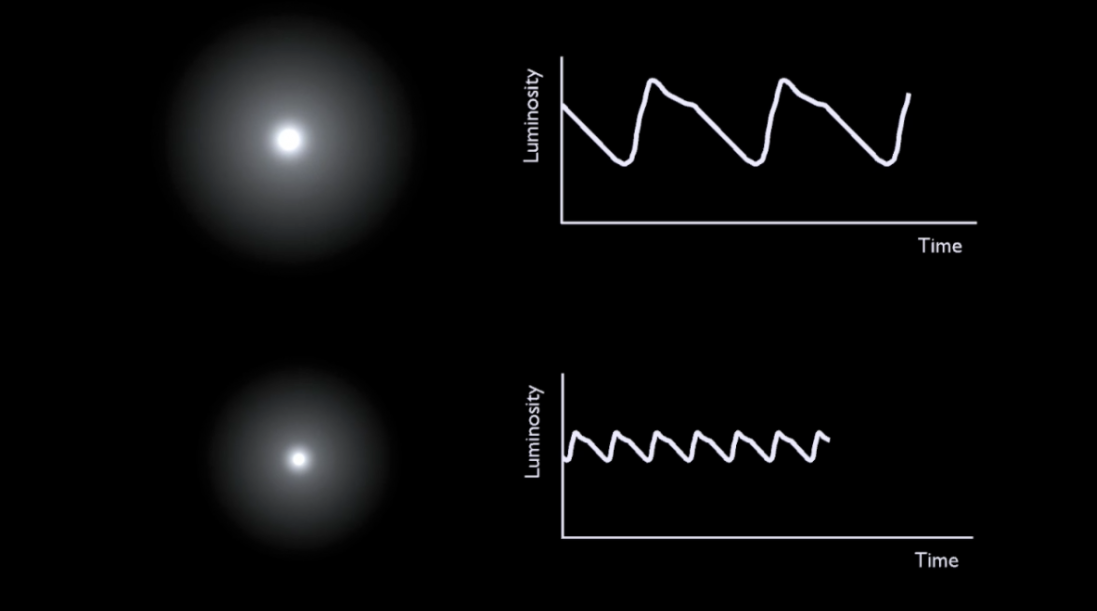We call this intrinsic brightness of the star absolute magnitude. And we define it as the brightness that the star would have if we placed it 10 parsecs away. On the contrary, the relative magnitude is the intensity with which each star shines at its distance from us.

HV 5497

I was looking for a Cepheid that is in my constellation, but I have not found any. In a document in which the constellation of Orion was mentioned, this Cepheid (HV 5497) appeared, but when I read the document more in depth I realized that it does not say that it is in Orion.
As I did not found any cepheids that belong to Orion, I decided to look for the period of HV 5497, which is 99 days.

Regression line:

I did this with the rextester program, which is an online code editor.
In the first row I changed the data for the 44 cepheids in the article by Lazovik et al, 2020. This row expresses the values ​​in days, and the second row is the sets of values ​​of magnitude.
Regressions, in this case, are logarithmic relations. In this program I ask it to print a summary of all the mathematical data in this relationship. I tell him what would happen if my value of days were exactly 99 days, that it would predict the result of my cepheid for me. We do this because we want to know with our own formula how much it corresponds in terms of its magnitude.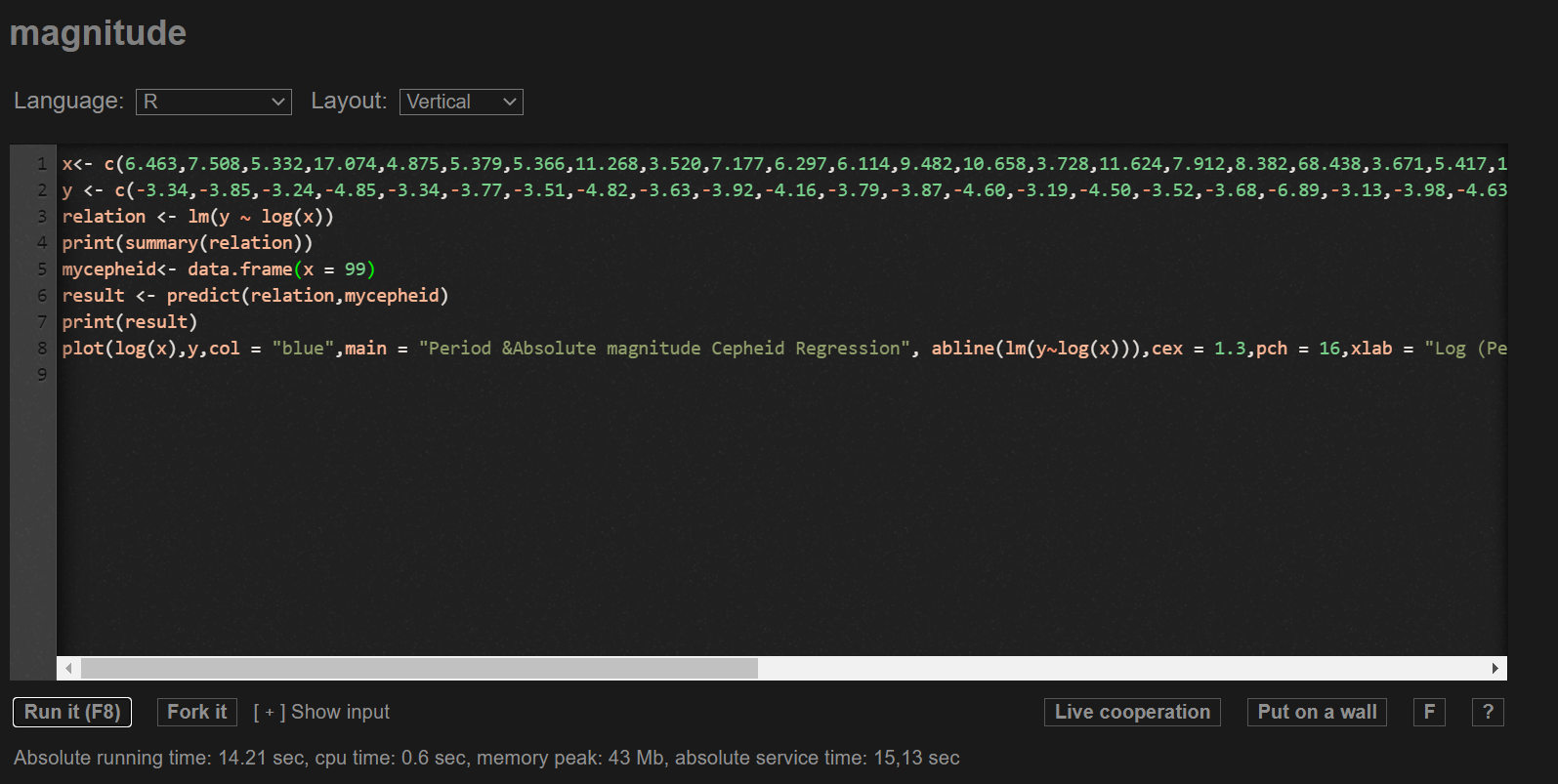In the following image we can see that it gives us the result of the interpolation of our period (in this case 99 days) and gives us the result of the magnitude it would have. In this case, it gives us that it has a magnitude of -6.8.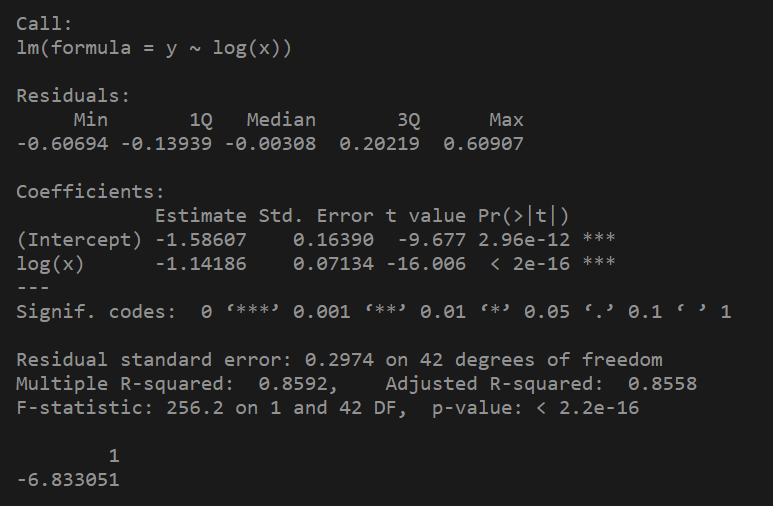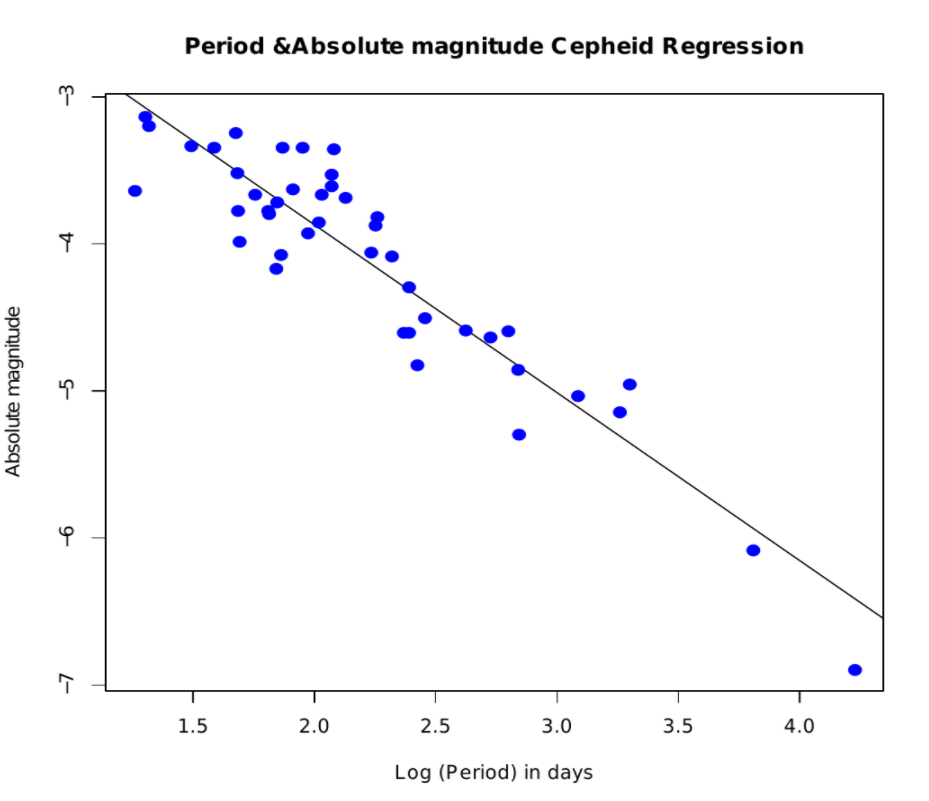------------------------------------------------------------------------------------------------------------------------------------

Also, I have made a graph, with Excel, in which you can see the relationship between magnitude and period of 505 Cepheids. From this, a formula has been created with which I can calculate the magnitude of a Cepheid from its period.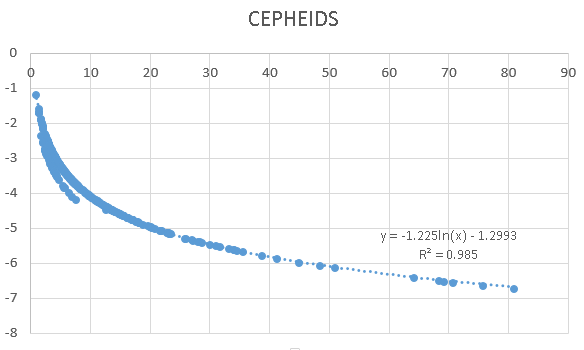I have calculated the magnitude from this formula obtained with Excel and I have also calculated the magnitude with the formula from ESA & ESO book.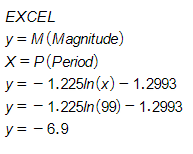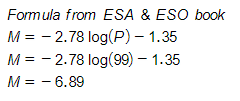We can see that the result of the first method and the two formulas is very similar.